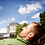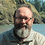This is an archived static version of the original phylobabble.org discussion site.

# Number of labeled histories given a topology?joaks

Is anyone aware of an algorithm for counting the number of labeled histories given a topology (with labeled tips)? It seems so obvious that it must exist, but the internet is failing me. I thought this would be easy to find in the coalescent literature, but the tips within populations are usually exchangeable (unlabeled) in this context. An existing implementation would be ideal. Thanks!felsenst

The total number of tree topologies for n tips for the rooted bifurcating case is known to be 1 x 2 x 3 x … x (2n-3) which is (2n-3)! / (2^(n-2) (n-2)!), and the number of bifurcating LH is n! (n-1)! / 2^(n-1) The ratio of those is n! (n-1)! (n-2)!/(2n-3)! which for n=3 is 1 per topology, which is right as considering all cases shows. However, we would need to know that different tree topologies each have the same number of LH, and that isn’t true as considering n=5 shows.

So, okay, I give up, but we at least can get a rough idea of the average number of LH per TT.max

I also happen to be interested in this question. @mathmomike, @ematsen and @cwhidden can you guys point us in the right direction here?mathmomike

If I understand your question correctly you have a leaf-labelled rooted phylogenetic tree (not necessarily binary) and you want to know how many ways there are to rank (i.e. order 1,2,3…) the interior vertices of the tree so that a vertex descended from another vertex always gets a lower number. For example when n=4 the binary caterpillar tree has only 1 ranking, while the balanced binary tree (‘fork’) has 2 rankings. Indeed there is an exact formula for this. Let I_V be the set of interior vertices of the tree, and for each interior v in I_V let l(v) be the number of interior vertices of T that are descendants of v (including v as a descendant of itself). Note that if T is binary then I_V has size n-1 (where n is the number of leaves of T) and l(v) is the number of leaves descended from v minus 1. The formula you want then for the number of rankings of T is I I_V| !/\prod_{v \in I_V} l(v).
For example, for the balanced binary tree with n=4, this gives 3!/(3x1x1) = 2. Hope that helps.max

I think that does it for me. Thanks a bunch, Mike!joaks

That’s exactly what I was looking for. Thanks!